# Simplifying FractionsProblem: Josephine ate four-eighths of a pie and Penelope ate six-twelfths of a pie. If both pies are the same size, then which girl ate more pie?

Analysis: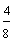?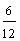We need to simplify these fractions in order to compare them more easily.

The numerator and denominator of a fraction are called its terms. If we simplify a fraction, then we are reducing it to lowest terms. Reducing a fraction to lowest terms will not change its value; the reduced fraction will be an equivalent fraction. All we need to do is divide the numerator and the denominator by the same nonzero whole number. This is shown below.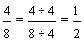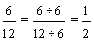Solution: Since four-eighths and six-twelfths can both be reduced to one-half, these fractions are equivalent. Therefore, Josephine and Penelope both ate the same amount of pie (one-half).=Dividing the numerator and the denominator of a fraction by the same nonzero whole number does not change the value of a fraction. This is because dividing by one does not change the value of a number.

Definition: A fraction is in lowest terms when the greatest common factor (GCF) of the numerator and denominator is 1.

To simplify a fraction (reduce it to lowest terms), the numerator and the denominator must be divided by the same nonzero whole number. Let's look at some examples of reducing a fraction to lowest terms.

Example 1: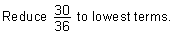Method 1: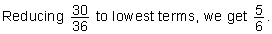With method 1, it can take several steps to reduce a fraction to lowest terms. Let's see what happens when we start by dividing the numerator and the denominator by their greatest common factor, instead of by their lowest common factor.

Example 1:Method 2: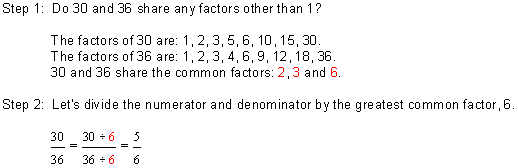With method 2, we found the greatest common factor of the numerator and the denominator. We then divided the numerator and denominator by their GCF. With this method it takes only two steps to reduce a fraction to lowest terms. So, method 2 is more efficient for reducing a fraction to lowest terms. The two methods for simplifying fractions are summarized below.

Reducing a Fraction to Lowest Terms (Simplest Form)

Method 1:

1. Find the common factors of the numerator and the denominator.
2. Divide the numerator and the denominator by their lowest common factor.
3. Find the common factors of the numerator and denominator in the resulting fraction.
4. Keep dividing by a common factor until there are no common factors other than 1

Method 2:

1. Find the GCF of the numerator and the denominator.
2. Divide the numerator and the denominator by their greatest common factor (GCF).

For the remainder of this lesson, we will use method 2 to reduce a fraction to lowest terms. Let's look at some more examples.

Example 2: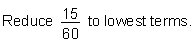Solution: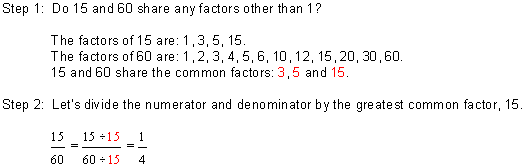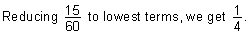Example 3:Solution: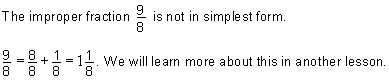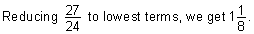Example 4: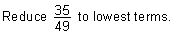Solution: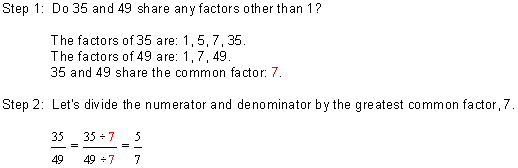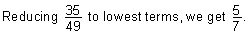Example 5:Solution: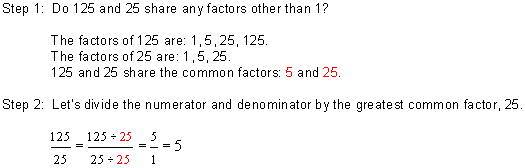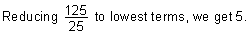Summary: To simplify a fraction (reduce it to lowest terms), the numerator and the denominator must be divided by the same nonzero whole number. A fraction is in lowest terms when the greatest common factor (GCF) of its numerator and denominator is one.

### Exercises

In Exercises 1 through 5, click once in an ANSWER BOX and type in your answer; then click ENTER. After you click ENTER, a message will appear in the RESULTS BOX to indicate whether your answer is correct or incorrect. To start over, click CLEAR. Note: To write the fraction two-thirds, enter 2/3 into the form.

 1.ANSWER BOX:   RESULTS BOX:
 2.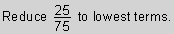ANSWER BOX:   RESULTS BOX:
 3.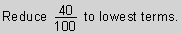ANSWER BOX:   RESULTS BOX:
 4.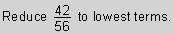ANSWER BOX:   RESULTS BOX:
 5.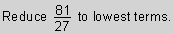ANSWER BOX:   RESULTS BOX:
 Lessons on Fractions 1. Introduction to Fractions 2. Classifying Fractions 3. Equivalent Fractions 4. Simplifying Fractions 5. Comparing Fractions 6. Ordering Fractions 7. Converting Fractions to Mixed Numbers 8. Converting Mixed Numbers to Fractions 9. Practice Exercises 10. Challenge Exercises 11. Solutions